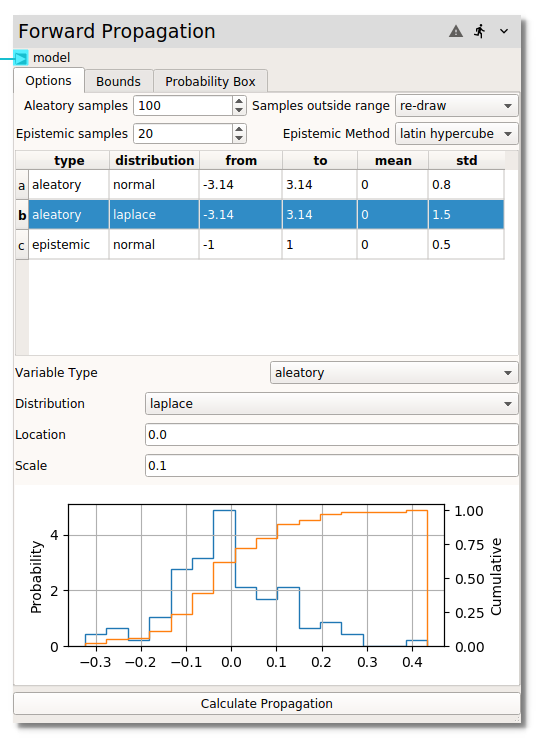# Forward Propagation¶

The Forward Propagation node allows for the exploration of aleatory variability and epistemic uncertainty of the model. Following the UQ methodology of Roy and Oberkampf [Roy2011], aleatory and epistemic uncertainties are segregated and aleatory variables are re-sampled for every epistemic sample. In the hybrid case, i.e., input variables are both aleatory and epistemic, the output model variability is expressed as a p-box or probability box. In the degenerate cases that only aleatory or epistemic variables are considered, the output will default to either a CDF or range (bounds).

## Options¶### global settings¶

Above the variable table are settings which are applied to all variables, most importantly the number of Aleatory samples and the number of Epistemic samples. When sampling aleatory variables from a distribution, especially when the number of samples becomes large, it is possible that input values will be drawn outside of the surrogate models’ support regions, i.e., values smaller or larger than the bounds used in the sampling design used for the construction of the surrogate. Three options are available for Samples outside range is detected:

• re-draw the full number of aleatory samples (for the given epistemic sample if considered)
• clip the sample to the extreme of the input variable range
• use the sample, even when it excedes the input variable range

Currently, a single sampling method is applied to all epistemic variables. The Epistemic Method dropdown provides all of the DOE Design options except CCD. The default LHS is strongly recommended for forward propagation sampling.

### variable specific¶

After selecting a varaible in the table, its specific options can be set below the table. First, the Variable Type is set to aleatory or epistemic. Aleatory variables need to have a specified Distribution :

• uniform
• normal
• lognormal
• gumbel
• laplace
• logistic
• wald

Alternatively, a user Distribution can be input in csv format. For epistemic variables, the only required input are the From and To bounds for sampling. Below the variable option specification area, an example distribution for the specified number of samples is provided. These samples are not directly used in the forward propagation step, but provide the user an idea of the spread which will be observed during sampling. If the range of the example sample significantly exceeds the limits of the input variables, this may result in:

• a quasi-infinite loop if Samples outside range are re-drawn
• a non-conservative estimation if Samples outside range are clipped
• a poor prediction if Samples outside range are used as-is

When variable specification is complete, the Calculate Propagation button at the bottom of the node (present in all tabs) is pressed to forward propagate the input uncertainties through the surrogate model to obtain the output uncertainties. If the propagation is successful, the output uncertainty will be displayed in the Probability Box tab. Otherwise, an error may be encountered if too many Samples outside range are encountered.## Bounds¶

When analyzing the (forwrd) propagated uncertainties, typical decision making questions are usually in the form of either:

• What is probability that the response will be above/below a certain reponse value?
• What is the response for a given probability, i.e., confidence level?

Either or both of these analyses can be performed on the p-box in the Bounds tab. In the former case, the desired response is entered in The probability that the value will be or less box. For hybrid input uncertainties, a probability range will be returned. In the latter case, as shown in the image below, the Given the prescribed input uncertainties with is set to 95 % probability. The example in the figure contains both types of uncertainties, therefore the response is returned as a range: the quantity of interest will be between 0.935 and 1.56. The response range at the 95% probability level is plotted on the p-box when the Draw on probability box is checked.

The Export bounds to file button allows the extremes of the p-box to be exported to a csv file.## Probability Box¶

The Probability Box tab displays the surrogate model propagated output uncertainty from the input variable uncertainties characterized in the Options tab. When both aleatory and epistemic uncertainties are present, each vector of aleatory uncertainties for a given epistemic sample is displayed as a cumulative distribution funtion (CDF) plotted as a thin black line. If the number of epistemic samples is high, this may simply appear as a dark region. The p-box is defined by the minimum and maximum response at each aleatory CDF level. The p-box bounds are highlighted in red and can be exported to a csv in the Bounds tab. The blue line represents a median distribution, i.e., if the hybrid aleatory-epistemic uncertainty segregation is removed and all data are collapsed into a single CDF. If response or probability bounds are set and displayed in the Bounds tab, they will appear on the p-box plot as dashed black lines.